# How did we use the + and - ends of resistors in this problem

## Homework Statement

https://6002x.mitx.mit.edu/static/circuits/ocwe1_1.png [Broken][/B]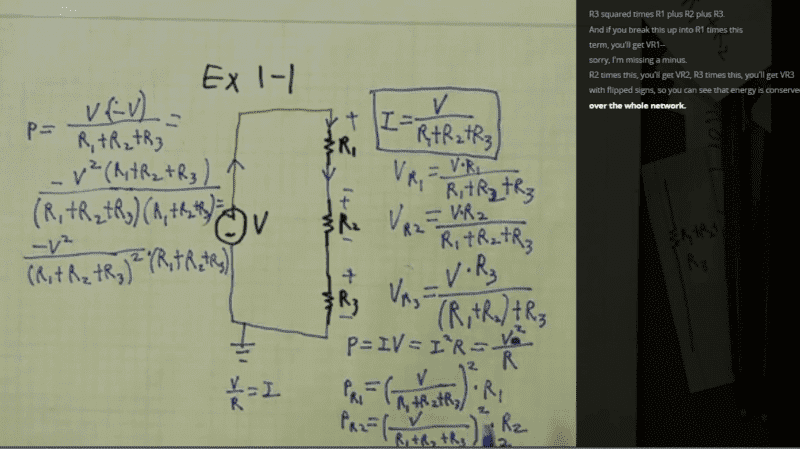What happens when you click on this link? https://6002x.mitx.mit.edu/section/three-series-resistors-slow/

Exercise 1-1, Part 1, Detail
Or this one
https://6002x.mitx.mit.edu/courseware/
The original problem is under Week 1 Tutorials

These are all publicly available videos and materials ^
I was able to view the above with just any computer

## Homework Equations

V=IR
P= I squared times V [/B]

## The Attempt at a Solution

[/B]
It's not my solution it is just the video walkthrough of the problem I have trouble with. The right hand side of the screenshot with handwriting is done before the power calculationso n the left.

In the video of the above, the speaker just labels the + and - ends of the resistors after having already computed V of R1, V of R2 etc. So he labeled them after doing the entire right side. He then says that this notation causes the negative sign in the Power equation. I don't understand that.

1. But first, I don't understand how the + and - markings on the resistors would affect any of the calculations on the right side. Once I understand that I think I will understand the negative sign on the power calculations better.

2. For the expression for I (the current) in the top right that is boxed in, does the markings of + or - on the resistors affect that expression?

3. What happens when you click on the links?

Last edited by a moderator:

NascentOxygen
Staff Emeritus
So he labeled them after doing the entire right side. He then says that this notation causes the negative sign in the Power equation.
The general form of the equation for electrical power into any element = Voltage across that element x current into that element

The loop current is marked, I. For that element on the left, its terminal voltage is V, and we can see its input current is -I, so its power input is V×(-I).

For the resistors, with loop current I already marked, you can determine the corresponding voltage & polarity across each individual resistor.

The sum of all the power inputs for all elements comes to zero.

Last edited:
CWatters
Homework Helper
Gold Member
Playing the devil... The resistors have +ve current going into the +ve terminal BUT -ve current going into the -ve terminal. Which is the "input current"?

The general form of the equation for electrical power into any element = Voltage across that element x current into that element

The loop current is marked, I. For that element on the left, its terminal voltage is V, and we can see its input current is -I, so its power input is V×(-I).

For the resistors, with loop current I already marked, you can determine the corresponding voltage & polarity across each individual resistor.

The sum of all the power inputs for all elements comes to zero.

For the bolded in the quote, what did we do to see that the input current is I?
For the underlined in the quote,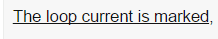as far as I can tell the only labeling of I is mentioning it under ground as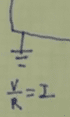without direction.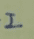as far as I can tell is just him making up a variable but I don't understand how it was originally defined to give us the signs we end up with.

The + and - on the resistors that the lecturer write also seems somewhat arbitrary to me given that there are no + and - in the original problem statement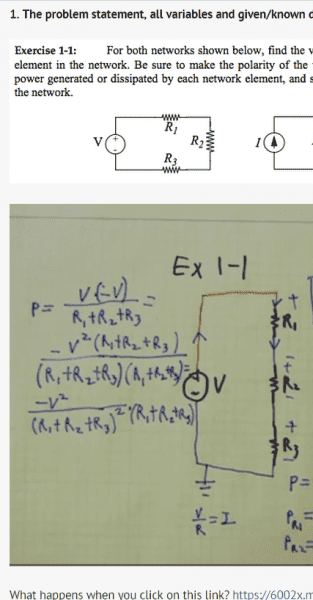NascentOxygen
Staff Emeritus